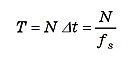FFT analyzer FAQThe relationship between time window length and number of sampling points.The number of finite points (number of FFT points) is shown here as number of sampling points. The FFT time window length is determined by both the number of sampling points and the sampling frequency (sampling interval). The relationship is found with the following equation if the number of sampling points is N, sampling interval is Δt(s), and the sampling frequency is fs(Hz)(1)

If the frequency range is 20 kHz, the sampling frequency is 51.2 kHz. And T = 2048/51.2 = 40(ms) when N = 2048 since the number of sampling points N is usually expressed as a power of 2 as described in the section on FFT.

Revised:2009/11/16